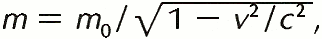Definition: mass from Dictionary of Energy

Physics. a fundamental property of an object that makes it resist acceleration, and that determines its gravitational attraction. This property can be generally regarded as equivalent to the amount of matter in the object. Mass and weight are not strictly synonymous; mass is not affected by the forces acting on an object, but weight is a relative property that can change according to the gravitational force exerted on the object.

Summary Article: mass, in physics
From The Columbia Encyclopedia

in physics, the quantity of matter in a body regardless of its volume or of any forces acting on it. The term should not be confused with weight, which is the measure of the force of gravity (see gravitation) acting on a body. Under ordinary conditions the mass of a body can be considered to be constant; its weight, however, is not constant, since the force of gravity varies from place to place. There are two ways of referring to mass, depending on the law of physics defining it: gravitational mass and inertial mass. The gravitational mass of a body may be determined by comparing the body on a beam balance with a set of standard masses; in this way the gravitational factor is eliminated. The inertial mass of a body is a measure of the body's resistance to acceleration by some external force. One body has twice as much inertial mass as another body if it offers twice as much force in opposition to the same acceleration. All evidence seems to indicate that the gravitational and inertial masses of a body are equal, as demanded by Einstein's equivalence principle of relativity; so that at the same location equal (inertial) masses have equal weights. Because the numerical value for the mass of a body is the same anywhere in the world, it is used as a basis of reference for many physical measurements, such as density and heat capacity. According to the special theory of relativity, mass is not strictly constant but increases with the speed according to the formulawhere m0 is the rest mass of the body, v is its speed, and c is the speed of light in vacuum. This increase in mass, however, does not become appreciable until very great speeds are reached. The rest mass of a body is its mass at zero velocity. The special theory of relativity also leads to the Einstein mass-energy relation, E=mc2, where E is the energy, and m and c are the (relativistic) mass and the speed of light, respectively. Because of this equivalence of mass and energy, the law of conservation of energy was extended to include mass as a form of energy.The Columbia Encyclopedia, © Columbia University Press 2018

### Related Articles

##### Full text Article mass
Britannica Concise Encyclopedia

Quantitative measure of inertia, or the resistance of a body to a change in motion. The greater the mass, the smaller is the change produced by an

##### Full text Article mass
The Penguin Dictionary of Physics

Newton (1687) defined mass as the quantity of matter of a body, expressed as the product of volume times density. For example, a ball of wool is...

##### Full text Article massless
Collins English Dictionary

adj 1 physics having no mass

See more from Credo